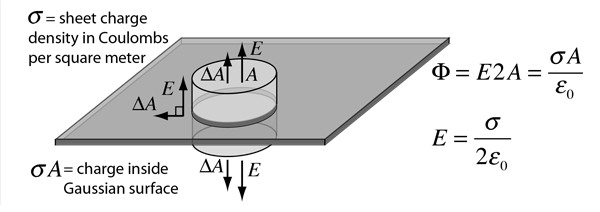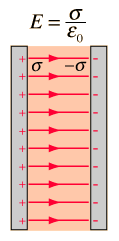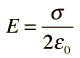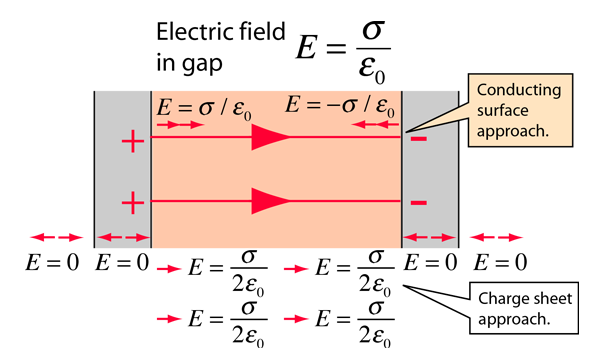Electric Field: Sheet of ChargeFor an infinite sheet of charge, the electric field will be perpendicular to the surface. Therefore only the ends of a cylindrical Gaussian surface will contribute to the electric flux . In this case a cylindrical Gaussian surface perpendicular to the charge sheet is used. The resulting field is half that of a conductor at equilibrium with this surface charge density.

 Fields for other charge geometries
Index

Electric field concepts

 HyperPhysics***** Electricity and Magnetism R Nave
Go Back

Electric Field: Parallel Plates

If oppositely charges parallel conducting plates are treated like infinite planes (neglecting fringing), then Gauss' law can be used to calculate the electric field between the plates. Presuming the plates to be at equilibrium with zero electric field inside the conductors, then the result from a charged conducting surface can be used:This is also consistent with treating the charge layers as two charge sheets with electric fieldin both directions.Fields for other charge geometries Application in capacitor
 Electric field and work for parallel plates
Index

Electric field concepts

 HyperPhysics***** Electricity and Magnetism R Nave
Go Back